# How many protons, electrons, and neutrons are in an atomPage 1

#### WATCH ALL SLIDES

Slide 1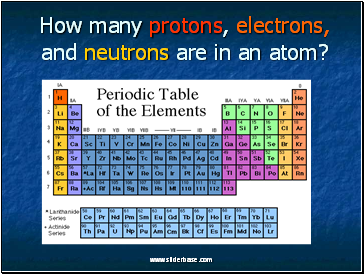## How many protons, electrons, and neutrons are in an atom?

Slide 2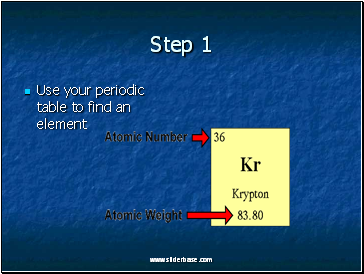Step 1

Use your periodic table to find an element

Slide 3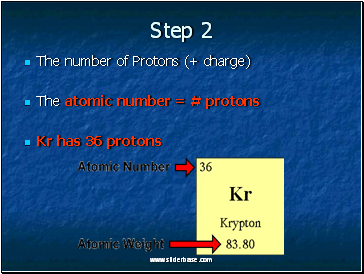Step 2

The number of Protons (+ charge)

The atomic number = # protons

Kr has 36 protons

Slide 4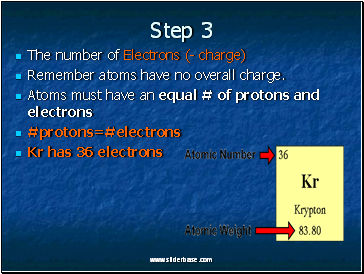Step 3

The number of Electrons (- charge)

Remember atoms have no overall charge.

Atoms must have an equal # of protons and electrons

#protons=#electrons

Kr has 36 electrons

Slide 5Step 4

The number of Neutrons (no charge)

Mass # (atomic weight rounded to nearest whole #)

Neutrons = Mass # - Protons

Neutrons = 84 – 36

Neutrons = 48

Slide 6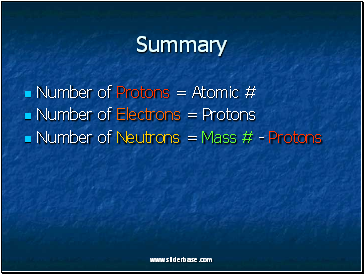Summary

Number of Protons = Atomic #

Number of Electrons = Protons

Number of Neutrons = Mass # - Protons

Slide 7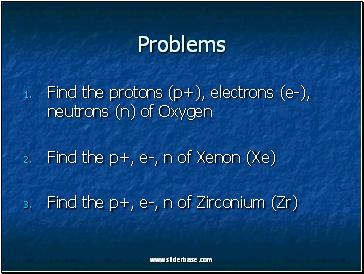## Problems

Find the protons (p+), electrons (e-), neutrons (n) of Oxygen

Find the p+, e-, n of Xenon (Xe)

Find the p+, e-, n of Zirconium (Zr)

Slide 8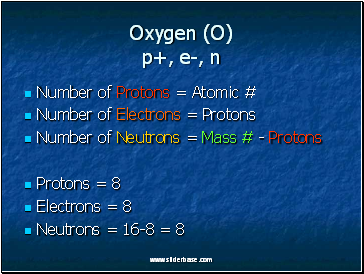Oxygen (O) p+, e-, n

Number of Protons = Atomic #

Number of Electrons = Protons

Number of Neutrons = Mass # - Protons

Protons = 8

Electrons = 8

Neutrons = 16-8 = 8

Slide 9Xenon (Xe) p+, e-, n

Number of Protons = Atomic #

Number of Electrons = Protons

Number of Neutrons = Mass # - Protons

Protons = 54

Electrons = 54

Neutrons = 131-54 = 77

Slide 10Zirconium (Zr) p+, e-, n

Number of Protons = Atomic #

Number of Electrons = Protons

Number of Neutrons = Mass # - Protons

Protons = 40

Electrons = 40

Neutrons = 91-40 = 51